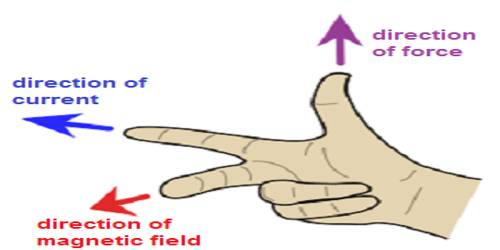# Determination of Magnitude and Direction of Magnetic Field

Determination of magnitude and direction of magnetic field

Magnetic field lines are some dosed curved lines drawn on magnetic field and tangents are drawn on them at any point gives the direction of magnetic field at that point.

Determination of magnitude: If a charge is in the motion perpendicular to the magnetic field and if the magnitude of the magnetic force acting on that charge is F, then the product of the charge and the force gives the magnitude of the magnetic field. Let q be the charge, v the velocity and F, the force, then the magnitude of magnetic field B is,

B = F / qv

In other words, we can say that if a unit charge moving perpendicular to the magnetic field with unit velocity, then the force experienced by the charge is equal to the magnitude of the magnetic field.

Determination of direction: If a magnetic needle is placed in a magnetic field, the direction of its north pole is the direction of the magnetic field.

From the above discussion, the following definition of magnetic field B can be given. The region around a current-carrying wire or a magnet in which a magnetic needle shows deflection is called the magnetic field of that magnet or the current-carrying wire.

If a unit charge moving perpendicular to the magnetic field with unit velocity, then the force experienced by the charge is equal to the magnitude of the magnetic field and if a magnetic needle is placed in the magnetic field the direction that its north pole indicates is the direction of the field.The direction of magnetic field produced by a wire carrying current is determined by the right-hand rule-2 (Figure). The role is as follows-

Fleming’s right-hand rule-

If a wire carrying current is grasped or held by right hand with the extending thumb pointing in the direction of the current, then the other fingers point the direction of the magnetic field (Figure). This rule is also known as right-hand rule-2. In order to determine the direction of magnetic force right-hand rule-1 is used.